# Percent practice

Percentage is a way of expressing a number, especially a ratio, as a fraction of 100. See also our verbal problems in percent or
examples1, examples2, examples3, examples4, examples5.

Calculate:
 Number of examples: Range of values: Complexity:
 26% of 96 = 17% of 19 = 71% of 93 = 46% of 26 = 70% of 57 = 92% of 16 = 35% of 65 = 84% of 6 = 98% of 19 = 1% of 17 =

### Percentage in word math problems

• A perineumA perineum string is 10% shorter than its original string. The first string is 24, what is the 9th string or term?
• Discount saleAt Christmas Sale after a 20% discount, the cosmetic package was priced for 5 euros and 60 cents; later it went to 3 euros and 80 cents. How much percentage is the total discount?
• WeightlifterWeightlifter lifted 75% of its weight. Determine how much weight lifted when he weighs 132 kg.
• Green - Red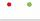We have 5 bags. Each consist one green and 2 red balls. From each we pull just one ball. What is the probability that we doesn't pull any green ball?
• Backpack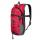Large backpack cost CZK 1352, little is 35% cheaper. How much we paid for 5 large and 2 small backpacks?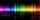The glass of 1 mm thickness absorb 4% of UV radiation passing through. How many percent of UV rays absorb glass with a thickness 1.1 cm, made from 1 mm thick those glasses?
• Sales taxA sales tax on a Php 10,800 appliance is Php.1,620. What is the rate of sales tax?
• VacationTomas spent 60% of his savings for his weekly vacation. He was 32 € left. How many euros did he have before vacation?
• Mini-surveyIn the mini-survey of our class about the popularity of individual subjects, it turned out that 11.1% of pupils like mathematics, 18.5% are enjoying languages, 30.4% of pupils like physical education, and the remaining 12 pupils have several popular subje
• Earth's surface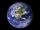The greater part of the earth's surface (r = 6371 km) is covered by oceans; their area is approximately 71% of the Earth's surface. What is the approximate area of the land?
• Accidents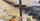The count of accidents in 1995 caused 8780 women which is 8.4%. How many men caused the accidents?
• MushroomsFresh mushrooms contain 88% water, dried 14% water. How many kilograms of fresh mushrooms should be collected to get 3 kg of dried?
• Nectar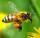Nectar collected by bees contains 70% water. From the nectar of the some process produces honey which contains 19% water. How many kg of nectar bees need to collect to make 1 kg of honey?